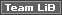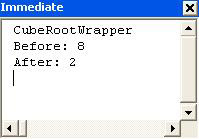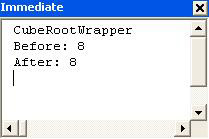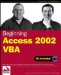# ArgumentsNormally, when an argument is passed to a function, it is passed by reference . In other words, the procedure receiving the argument is passed a reference, or pointer, which indicates where in memory the variable is stored. It doesn't receive the actual value of the variable.

Passing variables by reference has two effects. The advantage is that it's faster - Access doesn't need to make a copy of the value in another location ready for the procedure to read. However, because the procedure has access to the original variable (it knows where it is stored in memory), it can change the value of this variable. When the procedure ends, the code that called the procedure will see and work with the new value.

This is useful if you want the procedure to change the value, but it can create unexpected results if the value is changed when you have not planned it.

In VBA the default is to pass arguments by reference ( ByRef ), however, where applicable , we suggest that you consider passing arguments by value ( ByVal ). It is good programming practice, if the value is not to be changed to pass it by value. Then you know when debugging that it was not changed in that function. Generally developers new to programming seem to experience problems when passing by reference (such as unintentionally changing values of variables). Another reason why we favor passing arguments by value, where appropriate, is that it is the default in the new VB.NET language, and traditionally many Access VBA developers advance to develop VB applications (or now VB.NET applications). Of course, there may be times when you pass by reference, for example, passing an object by reference. We will see as we work through this chapter where we should pass ByRef and ByVal .

### Passing Arguments By Value

Our favored choice is to pass arguments by value. As we have said, this is where VBA just makes a copy of the data and passes that copy to the procedure. In this way, if the value of the copy is changed, the original value remains the same.

Let's compare the two methods by using each of them to calculate the cube root of 8.

### Try It Out-Passing Arguments By Reference and By Value

1. Open the Chapter 11 Code module and create the CubeRoot() subprocedure by typing in the following code:

`   Sub CubeRoot(dblNumber As Double)     dblNumber = dblNumber ^ (1 / 3)     End Sub   `
2. Now create a procedure which will call the CubeRoot() procedure:

`   Sub CubeRootWrapper()     Dim dblVariable As Double     dblVariable = 8     Debug.Print "Before: " & dblVariable     CubeRoot dblVariable     Debug.Print "After: " & dblVariable     End Sub   `
3. Run the CubeRootWrapper() procedure either by hitting F5 or by entering its name in the Immediate window and hitting Enter . You should then see the following in the Immediate window:4. Now modify the CubeRoot() subprocedure by inserting the keyword ByVal before the argument, like this:

`   Sub CubeRoot(ByVal dblNumber As Double)   dblNumber = dblNumber ^ (1 / 3) End Sub `
5. Run the CubeRootWrapper() procedure again. The output should now look like this:How It Works

The CubeRoot() subprocedure simply calculates the cube root of a number (no surprises there). The main procedure then prints the number twice - once before the subprocedure is run and once after.

By default, VBA passes variables by reference. Therefore, the first time we run the CubeRootWrapper() procedure, we pass the variable dblVariable to the CubeRoot() procedure by reference. In other words, the CubeRootWrapper() procedure passes a pointer to the place where dblVariable is stored in memory.

` CubeRoot dblVariable `

The CubeRoot() procedure labels what it finds at this location as dblNumber and then modifies the contents of that memory location.

` dblNumber = dblNumber ^ (1 / 3) `

Consequently, when the CubeRootWrapper inspects what is at that location, it finds that its contents have changed.

` Debug.Print "After: " & dblVariable `

We then change the code in CubeRoot() . Placing the ByVal keyword before the argument means that it will be passed by value instead of by reference - the actual value of the variable will be passed, as opposed to just a pointer to its memory address.

` Sub CubeRoot(ByVal dblNumber As Double) `

This time, the CubeRoot() procedure has no idea where the original variable dblVariable in the calling procedure is located in memory - all it has got is its value. The variables dblNumber and dblVariable are now quite distinct from each other. It quite happily changes the value of dblNumber , but dblVariable is not modified.

Passing arguments by reference is quicker than passing by value, as we noted earlier, but you should consider passing arguments by value, especially in the following circumstances:

• When you do not want the contents of a variable to change, but you need to pass it to a procedure that someone else has written and you don't know how it works. After all, you've no idea what the procedure you are calling might do to your variable!

• When passing variables to procedures in DLLs.

If you have no idea what that last point means, don't worry. That's what we are going to look at now - how to extend the functionality of VBA through using DLLs.Beginning Access 2002 VBA (Programmer to Programmer)
ISBN: 0764544020
EAN: 2147483647
Year: 2003
Pages: 256